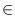Next: A Floating Point Filter Up: Number Types and Linear Previous: The mod kernel of   Contents   Index

# The smod kernel of type residual ( residual )

Definition

Type residual::smod is a variant of class residual::mod that uses a signed representation. Here numbers modulo p are represented by integral doubles in (- p/2, + p/2). All functions have the common precondition that p is a prime between 3 and 226. The functions of type residual::mod are also provided for class residual::smod and have the same meaning, so we do not list them separately here.

#include < LEDA/numbers/residual.h >

Operations

 double residual::frac(double a) returns a + z where z is the unique integer such that a + z[- 1/2, 1/2)

Creation

 residual x creates an instance x of type residual and initializes it with zero. residual x(long a) creates an instance x of type residual and initializes it with the value of a. residual x(int a) creates an instance x of type residual and initializes it with the value of a. residual x(double a) creates an instance x of type residual and initializes it with the integral part of x. residual x(const integer& a) creates an instance x of type residual and initializes it with the value of a.

Operations

 int residual::set_maximal_bit_length(int b, bool with_check=do_overflow_check) sets the maximal bit size of the representable numbers to b and returns the previous maximal bit size int residual::get_maximal_bit_length() returns the maximal bit size of the representable numbers int residual::required_primetable_size(int b) returns the number of primes required to represent signed numbers up to bit length b

The following functions have the common precondition that the residual objects a, x are integral and do not overflow.

 integer x.to_integer() returns the integer equal to x. long x.length() returns the length of the binary representation of the integer represented by x. bool x.is_long() returns true if and only if x fits in the data format long. long x.to_long() returns a long number which is initialized with the value of x. Precondition x.is_long() is true. double x.to_double() returns a double floating point approximation of x. double x.to_float() as above. bool x.is_zero() returns true if and only if x is equal to zero. bool x.is_invertible() returns true if and only if x is nonzero and the current modular representation of x allows to invert x without loss of information. int x.sign() returns the sign of x. int x.lagrange_sign() returns the sign of x using Lagrange's formula. int x.garner_sign() returns the sign of x using Garner's formula. string x.to_string() returns the decimal representation of x. residual abs(const residual& a) returns the absolute value of a void x.absolute(const residual& a) sets x to the absolute value of a.

The remaining functions do not have implicit preconditions. Although not explicitly mentioned, the arithmetic operations +, -, *, /, +=, -=, *=, /=, + +, - -, the shift operations, the comparison operations <, <=, >, >=, ==, != and the stream operations are available.

 residual sqr(const residual& a) returns a*a residual det2x2(const residual& a, const residual& b, const residual& c, const residual& d) returns a*d-b*c void x.add(const residual& a, const residual& b) sets x to a+b. void x.sub(const residual& a, const residual& b) sets x to a-b. void x.mul(const residual& a, const residual& b) sets x to a*b. void x.div(const residual& a, const residual& b) sets x to a/b. void x.det2x2(const residual& a, const residual& b, const residual& c, const residual& d) sets x to a*d-b*c. void x.inverse(const residual& a) sets x to the modular inverse of a. Precondition x.in_invertible is true. void x.negate(const residual& a) sets x to -a.

The following functions provide direct read-only access to the internal representation of residual objects. They should only be used by the experienced user after reading the full documentation of type residual.

 residual_sequence residual::get_primetable() returns a copy of the currently used primetable residual_sequence residual::get_garnertable() returns a copy of the currently used table of Garner's constants residual_sequence get_representation() returns a copy of the residual sequence representing xNext: A Floating Point Filter Up: Number Types and Linear Previous: The mod kernel of   Contents   Index